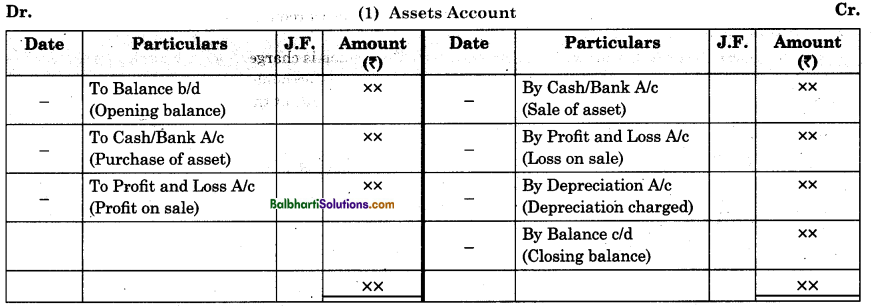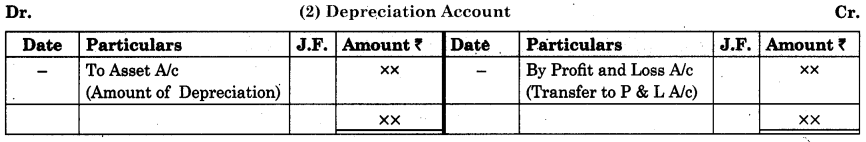By going through these Maharashtra State Board Bookkeeping and Accountancy 11th Notes Chapter 7 Depreciation students can recall all the concepts quickly.

## Maharashtra State Board 11th Accounts Notes Chapter 7 Depreciation

Meaning And Definition of the Term Depreciation-

When fixed assets are used for producing goods and services of a business, their values are bound to decrease. Such reduction in the value of fixed assets due to their productive use is called depreciation. The word ‘depreciation’ is derived from the Latin word ‘depretium’ which means ‘decline’ or ‘reduction’ in value of a fixed asset due to its natural wear and tear or any other similar causes.

In the words of well-known author Carter, “Depreciation is a gradual, continuous and permanent decrease in the value of the asset from any cause whatsoever according to William Pickles, “Depreciation is the gradual and permanent decrease in the value of an asset from the cause.” The following illustration will make the above idea clear. If the computer costs you ₹ 35,000 when you purchase it.

After using it for two years, if you want to sell it away, you will not get ₹ 35,000 but much less than its original price. Such reduction or loss in price whatsoever takes place and in accounting, the terminology is known as depreciation. Depreciation is always charged on fixed assets. It is a part of operating expenses. It is a reduction in the value of assets due to their productive uses, and such reduction is gradual, continuous, and permanent. Depreciation A/c is a nominal account. Depreciation is always charged to Profit and Loss A/c at the end of the accounting year to arrive at a correct net profit or net loss of business. .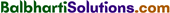Causes of Depreciation-

Causes of depreciation are explained below:

• Natural wear and tear and antiquity: As a result of use of assets during the course of business, their value gets reduced every year. Value of fixed assets does not remain same due to natural wear and tear and antiquity.
• Passage of effluxion of time: Assets get depreciated due to effluxion of time hence it is necessary to
depreciate them even if they are not in use.
• Obsolescence: On account of new inventions and technological development in asset or techniques of production, the old or existing assets become outdated, although they can be used. Such loss or reduction in the value of assets due to invention is called obsolescence.
• Natural calamities: Fall in the price of fixed asset may take place due to accidents like fire, earthquake, storm, cyclone, etc.
• Invention: When new machine or asset is invented, the earlier asset or machine in use may lose its utility and hence looses its value.
• Market value: The market price of an asset is also determined by its market demand and market supply. Market price of an asset goes on falling if its demand falls without corresponding fall in its supply.
• Depletion: To deplete means to empty. Depletion is also one of internal causes of decrease in value of wasting assets such as forests, oil wells, quarries, etc.Need And Importance of Depreciation-

1. To ascertain true and correct profit and loss: Depreciation reduces value of assets. It is an expense of the business. This operating expense is charged to Profit and Loss Ac. Unless depreciation is written off to Profit & Loss Ac, profit or loss whichever is shown by P/L Ac will not give, correct result.

2. To present a true financial position of business: A1 types of assets belonging to business are listed
and shown on the asset side of the balance sheet. Balance Sheet shows correct and true financial position of a business if assets are shown by deducting depreciation from its original value.

3. Replacement of assets: Every asset used in business has specific life. At the end of its life that asset is required to be replaced by new asset. If depreciation fund is created and accumulated by charging it to Profit & Loss A/c every year, it enables the business to replace asset at due time.

4. To compute correct tax liability: When depreciation is charged to Profit & Loss A/c correctly along
with other business expenses and losses, it will give correct result of profit or loss. This enables business to compute and pay correct tax on taxable profit. It is necessary to charge depreciation to comply with the provisions made in Companies Act and Income Tax Act.

Factors Affecting Depreciation-

The factors affecting depreciation are stated as follows:

(i) Cost of an Assets: Cost of asset is one of the important factors required to be considered for computation of depreciation. Cost of asset refers to the purchase price of asset and its incidental or installation charges. In short Cost of Asset = Purchase price of asset + its incidental or installation charges. Incidental or installation charges refer to all those expenses incurred by business in connection with purchase of asset i.e. transportation cost, custom duty, octroi, brokerage, coolie charges, cost of fixing asset in factory, electrification charges, etc., e.g. a company purchased a machinery for ₹ 18,500/- and spent ₹1,500/- for erection of machinery. In this case cost of machinery is (18,500 + 1,500) ₹ 20,000/-. There is a direct relation between cost of asset and depreciation chargeable on that asset per year.

(ii) Residual Value (or Estimated Scrap Value): This is another important factor considered for computation of depreciation on asset. Total amount whatsoever received by selling used or obsolete asset or its spare parts is called residual value or scrap value, e.g. a company purchased a machinery for ₹ 20,000 and used it for 9 years. Then company sold it away for ₹ 3,000/-. Here residual value is ₹ 3,000/-.

(iii) Estimated Life of an Asset: The specific period for which fixed assets give useful services to the business organisation is called estimated life of an assets e.g. a machinery may be useful to the business say for 10 years. After 10 years its replacement is necessary to carry on business further. Usually life of the fixed assets is determined by the experts in the respective field. Depreciation is calculated by dividing net cost of asset by the estimated life of the asset.

Formula For Computation of Depreciation-

For computation of depreciation on fixed asset, following factors are considered viz. (a) cost of asset (b) estimated life of an asset and (c) residual value of asset i.e. scrap value.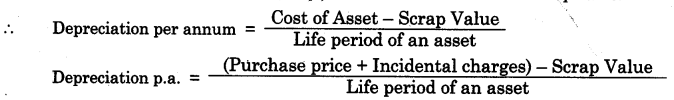(Where Cost of asset = Purchase price + Incidental charges.)
e.g. A Ltd. Co. purchased a machinery for ? 44,000/- and spent ? 1,000/- on its installation. Its estimated life is 10 years and its estimated residual value is ? 5,000/- work out depreciation chargeable per year.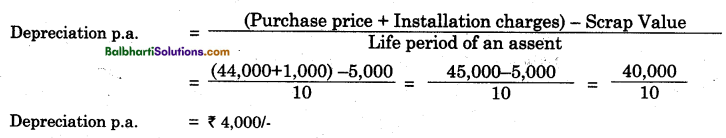Methods of Depreciation-

Depreciation on fixed asset may be charged by any one of the following given methods:

• Fixed Instalment Method
• Reducing Balance Method
• Annuity Method
• Depreciation Fund Method
• Insurance Policy Method
• Revaluation Method
• Machine Hour Rate Method etc.

(Only first two methods of depreciation are covered in this syllabus)

Fixed Instalment Method-

Meaning: This method of depreciation is also known as straight line method or original cost method or fixed percentage method of depreciation. This is one of the simplest method of depreciation. As name indicates, under this method depreciation at fixed percentage is computed on original cost of asset every year. According to this method, over a every full year period amount of depreciation and rate of depreciation remain same. This method of depreciation enables the business to spread depreciation evenly over a life period of asset.

Main features: Main features of fixed instalment method of depreciation are explained below :

• Depreciation is charged every year on original cost of asset.
• Amount of depreciation remains same over an every full year if there is no purchase or sale of assets.
• Rate of depreciation remains same every year.
• Amount of depreciation charged to Profit and Loss A/c remains identical during the economic life of the asset.

• It is simplest method of depreciation. Depreciation can be computed easily.
• Burden of depreciation amount spreads evenly over economic life of the assets.
• Book value of asset can be reduced to zero after its estimated useful life.
• If addition is made to existing assets with same life period, no separate calculations need to be made for ascertaining depreciation.

• If addition is made to existing assets which does not have same working life span, separate calculations for depreciation are required to be made for every new asset every time.
• In later life of asset, depreciation and repairs and renewal charges together give heavier burden on the business.
• This method of depreciation does not consider or take into account, interest chargeable on amount
invested in asset while charging depreciation on asset.

Reducing Balance Method-

Meaning: This method of depreciation is also known as written down value method or diminishing balance method of depreciation. It is a method of depreciation in which depreciation is computed at a fixed rate not on original cost of asset but on reducing balance of asset appearing at the beginning of each year. Under this method of depreciation, amount of depreciation goes on reducing year after year, but rate of depreciation remains fixed and same.

Main features : Main features of reducing balance method of depreciation are as follows:

• Depreciation is charged every year on opening cost of asset that appears in the beginning of the every year.
• Rate of depreciation charged, every year remains fixed and same.
• Amount of depreciation goes on reducing year after year.

• Under this method of depreciation, Profit and Loss A/c is debited uniformly for depreciation and repair charges every year in the sense that in the beginning depreciation amount chargeable is higher and repairs and renewal charges are lower. But in the later life of asset depreciation amount comes down with increase in the cost of repairs and renewals of asset.
• Under this method depreciation is charged on balancing cost of asset every year. Therefore there is no need to calculate depreciation separately on additions, if any, made.
• This method of depreciation is acceptable by business community as well as income-tax authority as
correct method of depreciation.

• As per this method of depreciation, interest on investment of asset made is altogether ignored while charging the depreciation.
• Value of asset cannot be brought down to zero even after its working life.
• If depreciation is considered for costing purpose, it costs higher in the beginning due to higher depreciation and in later life it costs lesser due to less amount of depreciation.

Illustrations: The following illustration will explain difference between fixed instalment method of depreciation and reducing balance method of depreciation. Let us presume that cost of machinery is ? 1,00,000/-. Rate of depreciation chargeable is 10% p.a.

 Year Fixed Instalment Method Reducing Balance Method I ₹ 10,000/- (10% of 1,00,000) ₹ 10,000 (10% of 1,00,000) II ₹ 10,000/- (10% of 1,00,000) ₹ 9,000 (10% of 90,000) III ₹ 10,000/- (10% of 1,00,000) ₹ 8,100 (10% of 81,000) IV ₹ 10,000/- (10% of 1,00,000) ₹ 7,290 (10% of 72,900)                  ‘ V ₹ 10,000/- (10% of 1,00,000) i.e. 10% on the original cost ₹ 6,561 (10% of 65,610) i.e. 10% on the balance.

Difference Between Fixed Instalment Method And Reducing Balance Method-

Fixed Instalment Method:

1. Meaning: Method of depreciation in which depreciation at fixed percentage (rate) is charged every year on original cost of fixed asset is called ‘Fixed Instalment Method’.
2. Amount of depreciation: Unless there is addition on or sale of fixed assets, the amount of depreciation remains same (constant) every year.
3. Suitability: This method of depreciation is easy to calculate and more suitable for the assets of small value and does not require to spent any amount on repairs and maintenance.
4. Acceptance of method: This method of depreciation is not accepted for income tax purposes.
5. Charge: Depreciation is charged on the original cost of asset every year.
6. Book value: After certain year book value of the asset becomes zero.

Reducing Balance Method:

1. Method of depreciation in which depreciation at fixed percentage (rate) is charged every year on opening cost of fixed asset is called ‘Reducing Balance Method’.
2. Unless there is any addition to or sale of fixed asset, the amount of depreciation goes on reducing every year.
3. This method of depreciation is difficult to calculate and more suitable for the assets of higher value having longer life requiring heavy expenditure in later life of assets.
4. This method of depreciation is accepted for calculation and payment of income tax.
5. Depreciation is charged on written down value of asset every year.
6. The book value of asset never becomes zero.Accounting Treatment of Depreciation-

(A) Journal entries in the books of firm.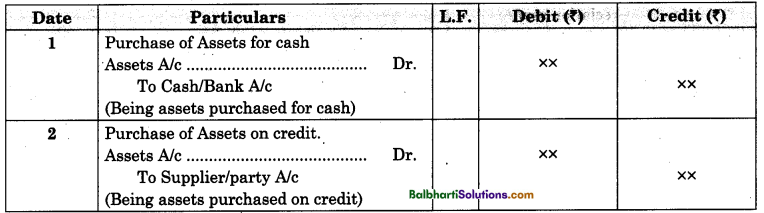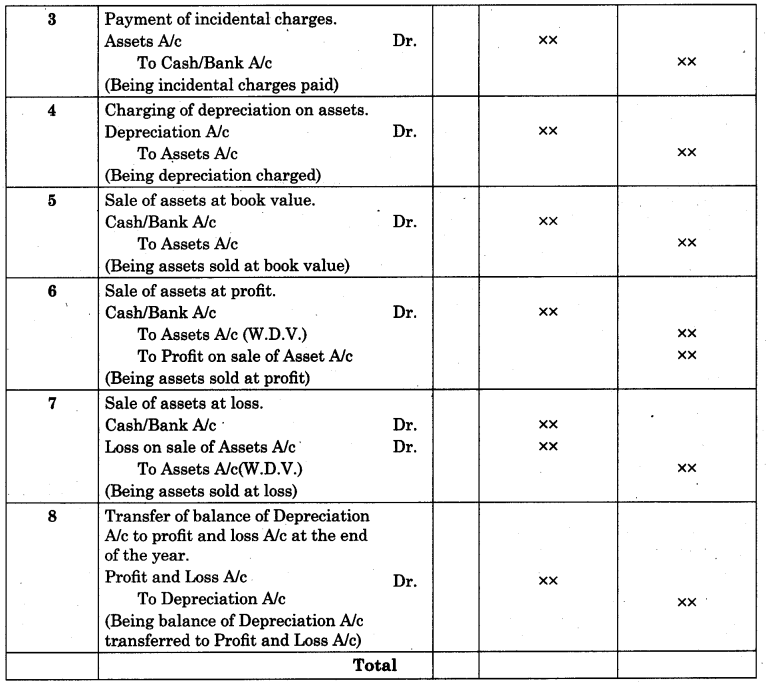(B) Ledger Accounts in the books of firm